Next: 4. Distributed Diagonaliser and Up: castep_performance_xt Previous: 2. Castep Performance on   Contents

Subsections

3.1 Introduction

The first stage of the project is to implement the basic band-parallelism. This involves distributing the wavefunction coefficients over thebands, in addition to the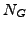G-vectors andk-points, as well as distributing other data such as the eigenvalues, band occupancies, and band-overlap matrices such as the subspace Hamiltonian. Along with the data distribution, as much of the workload as possible should also be distributed over the bands.

3.2 Programming

The main programming effort lies in the basic band-parallelism, and in particular the construction of band-overlap matrices which will now need to be distributed. Most of this effort will be concentrated in the wave module, which handles the vast majority of operations on wavefunctions and bands, but inevitably there are some changes required to other modules.

and a two-core band-parallel calculation produces

------------------------------------------------------------------------ <-- SCF
SCF loop      Energy           Fermi           Energy gain       Timer   <-- SCF
energy          per atom          (sec)   <-- SCF
------------------------------------------------------------------------ <-- SCF
Initial   5.10476316E+002  4.62264099E+001                        16.67  <-- SCF
1  -7.76802126E+002  2.64224391E+000   1.60909805E+002      28.13  <-- SCF
2  -8.50574887E+002  2.02770490E-001   9.22159500E+000      38.62  <-- SCF
3  -8.54801574E+002  3.79693598E-001   5.28335886E-001      52.16  <-- SCF
4  -8.52981743E+002  7.44988320E-001  -2.27478843E-001      60.98  <-- SCF
5  -8.52884167E+002  9.08590414E-001  -1.21969434E-002      70.63  <-- SCF
6  -8.52886334E+002  8.98636611E-001   2.70796284E-004      79.96  <-- SCF
7  -8.52887081E+002  9.06719344E-001   9.34638588E-005      88.78  <-- SCF
8  -8.52887250E+002  9.10591664E-001   2.11356795E-005      97.65  <-- SCF
9  -8.52887250E+002  9.11100143E-001  -3.08962712E-008     102.84  <-- SCF
10  -8.52887250E+002  9.11105407E-001  -4.65248977E-008     108.05  <-- SCF
11  -8.52887250E+002  9.11110563E-001  -1.77844503E-008     113.57  <-- SCF
------------------------------------------------------------------------ <-- SCF


Note that the results as reported are identical for the first 9 SCF cycles, and only differ by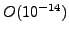eV/atom in the last two cycles, which is the same order as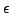for double-precision arithmetic and so may be attributed to different rounding errors for the serial and band-parallel calculations.

This calculation takes longer when run band-parallel compared to the serial calculation, but this is not a cause for alarm - the test system is very small, containing only 16 valence bands, so it is not surprising that the communication overhead outweighs the gains.

The same calculation run using the all-bands' self-consistent code path yields

------------------------------------------------------------------------ <-- SCF
SCF loop      Energy                           Energy gain       Timer   <-- SCF
per atom          (sec)   <-- SCF
------------------------------------------------------------------------ <-- SCF
Initial   6.83465549E+002                                         10.35  <-- SCF
1  -7.97053977E+002                    1.85064941E+002      20.90  <-- SCF
2  -8.48247959E+002                    6.39924773E+000      31.57  <-- SCF
3  -8.50914193E+002                    3.33279207E-001      42.16  <-- SCF
4  -8.51618587E+002                    8.80493249E-002      54.31  <-- SCF
5  -8.52080365E+002                    5.77221874E-002      64.82  <-- SCF
6  -8.52436527E+002                    4.45203123E-002      75.48  <-- SCF
7  -8.52663071E+002                    2.83179709E-002      85.99  <-- SCF
8  -8.52769350E+002                    1.32848145E-002      96.64  <-- SCF
9  -8.52812636E+002                    5.41075552E-003     107.15  <-- SCF
10  -8.52829576E+002                    2.11747553E-003     117.69  <-- SCF
11  -8.52836183E+002                    8.25924796E-004     128.48  <-- SCF
------------------------------------------------------------------------ <-- SCF


in serial, and

------------------------------------------------------------------------ <-- SCF
SCF loop      Energy                           Energy gain       Timer   <-- SCF
per atom          (sec)   <-- SCF
------------------------------------------------------------------------ <-- SCF
Initial   6.83465549E+002                                          7.04  <-- SCF
1  -7.97053977E+002                    1.85064941E+002      15.95  <-- SCF
2  -8.48247959E+002                    6.39924773E+000      24.90  <-- SCF
3  -8.50914193E+002                    3.33279207E-001      33.79  <-- SCF
4  -8.51618587E+002                    8.80493249E-002      43.01  <-- SCF
5  -8.52080365E+002                    5.77221874E-002      51.94  <-- SCF
6  -8.52436527E+002                    4.45203123E-002      61.04  <-- SCF
7  -8.52663071E+002                    2.83179709E-002      69.93  <-- SCF
8  -8.52769350E+002                    1.32848145E-002      79.12  <-- SCF
9  -8.52812636E+002                    5.41075552E-003      87.98  <-- SCF
10  -8.52829576E+002                    2.11747553E-003      96.76  <-- SCF
11  -8.52836183E+002                    8.25924796E-004     107.40  <-- SCF
------------------------------------------------------------------------ <-- SCF


in two-core band-parallel. Note that this time there is a small speed improvement for the band-parallel run - this is because the all-bands' path does more FFTs per SCF cycle than the DM path, and the FFTs distribute trivially among the band-group.

With the basic band-parallelism tested and complete, Castep has been demonstrated to work in band-parallel mode for the EDFT and DM algorithms.

The only known problem outstanding is with the EDFT mode. In the EDFT algorithm the empty bands are optimised non-self-consistently after the full bands have been updated, but at the moment this does not use the same algorithm as the DM code path and so is not band-parallel.

3.3 Benchmarking and Performance

The first reasonable simulation we performed with the new band-parallelism was the al1x1 benchmark. The test simulations were restricted to small test cases (8-atom silicon, and the al1x1 benchmark) and numbers of cores (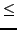8), where the results could be compared in detail to known results and serial calculations, but once testing was complete we were able to move to larger systems. Table 3.1 shows the performance improvement for the al1x1 benchmark using the new band-parallel mode.

Table 3.1: Parallel scaling of the al1x1 benchmark in band-parallel mode.
 cores DM efficiency 2 65% 4 50% 8 35%

Table 3.2: Execution time and parallel efficiency for the 33-atom TiN benchmark (8 k-points). Times are for 40 SCF cycles using the DM algorithm. The 8-core calculation is running purely k-point parallel, the others are running with mixed band and k-point parallelism.
 cores Time (s) band-parallel efficiency 8 5085.04 (k-point parallel) 16 3506.66 72% 32 2469.84 51%

The performance was analysed using Cray's Performance Analysis Tool (PAT) version 4.2. It was also necessary to create symbolic links to the Castep source files in Source/Utility in Castep's obj/linux_x86_64_pathscale/Utility and similarly for Fundamental and Functional.

pat_build -D trace-max=2048 -u -g mpi,blas,lapack,math castep


We profiled a Castep calculation on the al1x1 benchmark parallelised over 16 nodes (4-way band-parallel, 4-way gv-parallel). The subroutine with the most overhead from the band-parallelism was wave_rotate, the Trace output of which was:

|   o-> wave_orthonormalise_over_slice         1290                           |
|   o-> electronic_find_eigenslice             2580                           |
|   o-> wave_rotate_slice                      3870        3870     40.06s    |
|   o-> wave_nullify_slice                     3870        3870      0.01s    |
|   o-> wave_allocate_slice                    3870        3870      0.01s    |
|   o-> wave_initialise_slice                  3870        3870      1.75s    |
|   o-> comms_reduce_bnd_logical               3870        3870      0.28s    |
|   o-> comms_reduce_bnd_integer               3870        3870      0.10s    |
|   o-> comms_send_complex                    23220       23220      6.48s    |
|   o-> comms_recv_complex                    23220       23220      7.79s    |
|   o-> wave_copy_slice_slice                  3870        3870      1.09s    |
|   o-> wave_deallocate_slice                  3870        3870      0.00s    |


This is to be expected, since these wavefunction rotations scale cubically with system size, and also incur a communication cost when run band-parallel. Some time was spent optimising this subroutine, and in the end we settled on a refactoring of the communications whereby each node does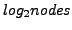communication phases, the first phase involving an exchange of half the transformed data, and each subsequent phase exchanging half the data of the previous one. This scheme is illustrated in figure 3.1.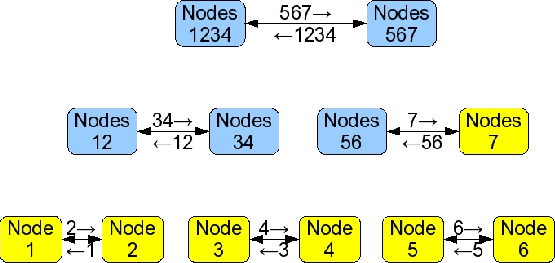This communication pattern improved the speed of the wave_rotate subroutine considerably, but at the cost of increased storage. Indeed the first phase involves the exchange of half of the newly transformed data, so the send and receive buffers constitute an entire non-distributed wavefunction. As the band-parallelisation is only efficient over relatively small numbers of nodes (typically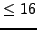) this has not proved too much of a hindrance thus far, but it would be wise to restrict this in future, perhaps to a low multiple of a single node's storage, at the cost of slightly more communication phases. Such a change could, of course, be made contingent on the value of the opt_strategy_bias parameter.

Once wave_rotate had been optimised, Castep's performance was measured on the al3x3 benchmark. As can be seen from figure 3.2, the basic band-parallelism implemented in this stage of the project improved Castep's scaling considerably. Using linear interpolation of the data points we estimated that the maximum number of PEs that can be used with 50% or greater efficiency has been increased from about 221 to about 436 (without using the SMP optimisations).

 [Castep performance]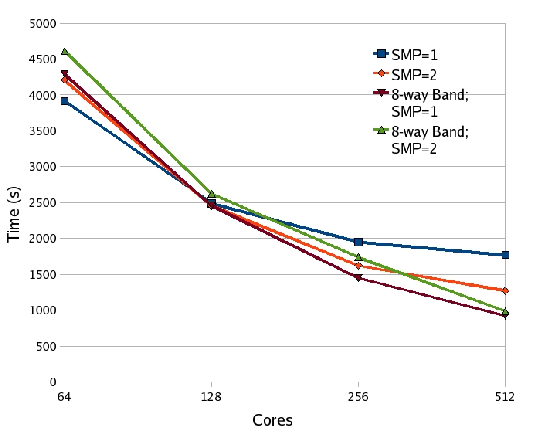[Castep parallel efficiency relative to 16 PE Castep 4.2]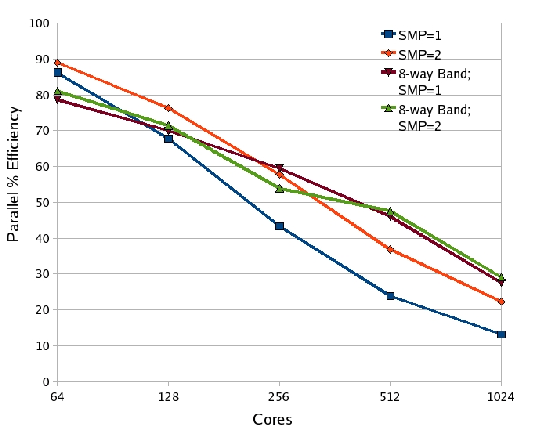Next: 4. Distributed Diagonaliser and Up: castep_performance_xt Previous: 2. Castep Performance on   Contents